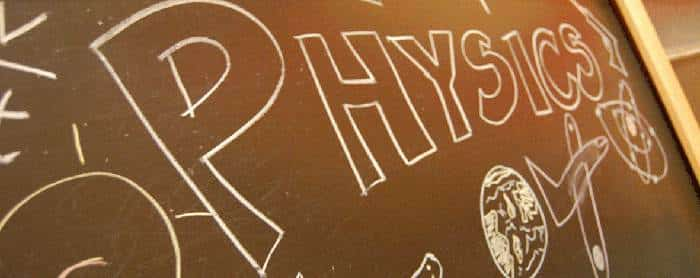# Multiple choice questions on Pressure and Energy### Energy and Pressure Physics MCQs:

Ques: An ideal gas is filled in a vessel, then
(a) If it is placed inside a moving train, its temperature increases
(b) Its centre of mass moves randomly
(c) Its temperature remains constant in a moving car
(d) None of these

Ans. (c)

Ques: In kinetic theory of gases, which of the following statements regarding elastic collisions of the molecules is wrong
(a) Kinetic energy is lost in collisions
(b) Kinetic energy remains constant in collision
(c) Momentum is conserved in collision
(d) Pressure of the gas remains constant in collisions

Ans. (a)

Related: MET Mathematics Practice Papers

Ques: The average kinetic energy of hydrogen molecules at 300 K is E. At the same temperature, the average kinetic energy of oxygen molecules will be
(a) E/4
(b) E/16
(c) E
(d) 4 E

Ans. (c)

Ques: Vapour is injected at a uniform rate in a closed vessel which was initially evacuated. The pressure in the vessel
(a) Increase continuously
(b) Decreases continuously
(c) First increases and then decreases
(d) First increase and then becomes constant

Ans. (c)

Ques: On colliding in a closed container the gas molecules
(a) Transfer momentum to the walls
(b) Momentum becomes zero
(c) Move in opposite directions
(d) Perform Brownian motion

Ans. (a)

Ques: The molecules of an ideal gas at a certain temperature have
(a) Only potential energy
(b) Only kinetic energy
(c) Potential and kinetic energy both
(d) None of the above

Ans. (b)

Ques: Two gases are at absolute temperatures 300 K and 350 K respectively. Ratio of average kinetic energy of their molecules is
(a) 7 : 6
(b) 6 : 7
(c) 36 : 49
(d) 49 : 36

Ans. (b)

Ques: The temperature of a gas is – 68°C. At what temperature will the average kinetic energy of its molecules be twice that of at – 68°C
(a) 137°C
(b) 127°C
(c) 100°C
(d) 105°C

Ans. (a)

Ques: The average translational kinetic energy of O2 (molar mass 32) molecules at a particular temperature is 0.048 eV. The translational kinetic energy of N2 (molar mass 28) molecules in eV at the same temperature is
(a) 0.0015
(b) 0.003
(c) 0.048
(d) 0.768

Ans. (c)

Ques: Vessel A is filled with hydrogen while vessel B, whose volume is twice that of A, is filled with the same mass of oxygen at the same temperature. The ratio of the mean kinetic energies of hydrogen and oxygen is
(a) 16 : 1
(b) 1 : 8
(c) 8 : 1
(d) 1 : 1

Ans. (d)

Ques: On absolute temperature, the kinetic energy of the molecules
(a) Becomes zero
(b) Becomes maximum
(c) Becomes minimum
(d) Remains constant

Ans. (a)

Ques: At the same temperature and pressure and volume of two gases, which of the following quantities is constant
(a) Total number of molecules
(b) Average kinetic energy
(c) Root mean square velocity
(d) Mean free path

Ans. (b)

Ques: The ratio of mean kinetic energy of hydrogen and oxygen at a given temperature is
(a) 1 : 16
(b) 1 : 8
(c) 1 : 4
(d) 1 : 1

Ans. (d)

Ques: The value of universal gas constant is 8.3 J/mole/K, the mean kinetic energy of 32 gm of oxygen at – 73°C will be
(a) 480 J
(b) 4980 J
(c) 2490 J
(d) The information is incomplete

Ans. (c)

Ques: The average kinetic energy of a gas molecule can be determined by knowing
(a) The number of molecules in the gas
(b) The pressure of the gas only
(c) The temperature of the gas only
(d) None of the above is enough by itself

Ans. (c)

Ques: The mean kinetic energy of a gas at 300 K is 100 J. The mean energy of the gas at 450 K is equal to
(a) 100 J
(b) 3000 J
(c) 450 J
(d) 150 J

Ans. (d)

Ques: The time average of the kinetic energy of one molecule of a gas taken over a long period of time
(a) Is proportional to the square root of the absolute temperature of the gas
(b) Is proportional to the absolute temperature of the gas
(c) Is proportional to the square of the absolute temperature of the gas
(d) Does not depend upon the absolute temperature of the gas

Ans. (b)

Ques: In kinetic theory of gases, a molecule of mass m of an ideal gas collides with a wall of vessel with velocity V. The change in the linear momentum of the molecule is
(a) 2mV
(b) mV
(c) – mV
(d) Zero

Ans. (a)

Ques: The pressure exerted by the gas on the walls of the container because
(a) It loses kinetic energy
(b) It sticks with the walls
(c) On collision with the walls there is a change in momentum
(d) It is accelerated towards the walls

Ans. (c)